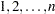OUTPUT Statement
OUTPUT OUT=SAS-data-set <DATA=SAS-data-set> <factor-value-settings> ;

The OUTPUT statement applies only to the last plan generated. If you use PROC PLAN interactively, the OUTPUT statement for a given plan must be immediately preceded by the FACTORS statement (and the TREATMENTS statement, if appropriate) for the plan.

You can specify the following options in the OUTPUT statement:

OUT=SAS-data-set
DATA=SAS-data-set

You can use the OUTPUT statement both to output the last plan generated and to use the last plan generated to randomize another SAS data set.

When you specify only the OUT= option in the OUTPUT statement, PROC PLAN saves the last plan generated to the specified data set. The output data set contains one variable for each factor in the plan and one observation for each cell in the plan. The value of a variable in a given observation is the level of the corresponding factor for that cell. The OUT= option is required.

When you specify both the DATA= and OUT= options in the OUTPUT statement, then PROC PLAN uses the last plan generated to randomize the input data set (DATA=), saving the results to the output data set (OUT=). The output data set has the same form as the input data set but has modified values for the variables that correspond to factors (see the section Output Data Sets for details). Values for variables not corresponding to factors are transferred without change.

factor-value-settings

specify the values input or output for the factors in the design. The form for factor-value-settings is different when only an OUT= data set is specified and when both OUT= and DATA= data sets are specified.

Both forms are discussed in the following section.

### Factor-Value-Settings with Only an OUT= Data Set

When you specify only an OUT= data set, the form for each factor-value-setting specification is one of the following:

factor-name <NVALS=list-of--numbers> <ORDERED | RANDOM> ;

or

factor-name <CVALS=list-of--strings> <ORDERED | RANDOM> ;

where

factor-name

is a factor in the last FACTORS statement preceding the OUTPUT statement.

NVALS=

listsnumeric values for the factor. By default, the procedure uses NVALS=(1 2 3).

CVALS=

listscharacter strings for the factor. Each string can have up to 40 characters, and each string must be enclosed in quotes. Warning: When you use the CVALS= option, the variable created in the output data set has a length equal to the length of the longest string given as a value; shorter strings are padded with trailing blanks. For example, the values output for the first level of a two-level factor with the following two different specifications are not the same.

```CVALS=('String 1' "String 2")

CVALS=('String 1' "A longer string")
```

The value output with the second specification is ’String 1’ followed by seven blanks. In order to match two such values (for example, when merging two plans), you must use the TRIM function in the DATA step (see SAS Language Reference: Dictionary).

ORDERED | RANDOM

specifies how values (those given with the NVALS= or CVALS= option, or the default values) are associated with the levels of a factor (the integers). The default association type is ORDERED, for which the first value specified is output for a factor level setting of 1, the second value specified is output for a level of 2, and so on. You can also specify an association type of RANDOM, for which the levels are associated with the values in a random order. Specifying RANDOM is useful for randomizing crossed experiments (see the section Randomizing Designs).

The following statements give an example of using the OUTPUT statement with only an OUT= data set and with both the NVALS= and CVALS= specifications.

```proc plan;
factors a=6 ordered b=3;
output out=design a nvals=(10 to 60 by 10)
b cvals=('HSX' 'SB2' 'DNY');
run;
```

The DESIGN data set contains two variables, a and b. The values of the variable a are 10 when factor a equals 1, 20 when factor a equals 2, and so on. Values of the variable b are ‘HSX’ when factor b equals 1, ‘SB2’ when factor b equals 2, and ‘DNY’ when factor b equals 3.

### Factor-Value-Settings with OUT= and DATA= Data Sets

If you specify an input data set with DATA=, then PROC PLAN assumes that each factor in the last plan generated corresponds to a variable in the input set. If the variable name is different from the name of the factor to which it corresponds, the two can be associated in the values specification by

input-variable-name = factor-name ;

Then, the NVALS= or CVALS= specification can be used. The values given by NVALS= or CVALS= specify the input values as well as the output values for the corresponding variable.

Since the procedure assumes that the collection of input factor values constitutes a plan position description (see the section Output Data Sets), the values must correspond to integers less than or equal to, the number of values selected for the associated factor. If any input values do not correspond, then the collection does not define a plan position, and the corresponding observation is output without changing the values of any of the factor variables.

The following statements demonstrate the use of factor-value-settings. The input SAS data set a contains variables Block and Plot, which are renamed Day and Hour, respectively.

```proc plan;
factors Day=7 Hour=6;
output data=a out=b
Block = Day  cvals=('Mon' 'Tue' 'Wed' 'Thu'
'Fri' 'Sat' 'Sun'      )
Plot  = Hour;
run;
```

For another example of using both a DATA= and OUT= data set, see the section Randomly Assigning Subjects to Treatments.